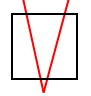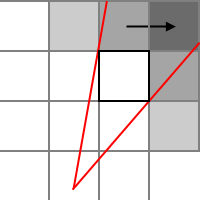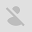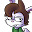# Erik McClure

#### Tile-Based Discrete Wavefront Propagation

I'm currently building a very simplistic first-person shooter in WebGL. An example of this algorithm, with the debug code left in, is available here. The map is represented by a grid - a cell is solid if its 0, and empty if its 1. This is trivial to render by using a standard recursive 4-direction flood fill algorithm. Unfortunately, we can't simply render the entire level if our rooms contain high levels of detail or many objects, because we'll overload the GPU.

Frustum culling is the obvious answer, but I need it to be highly efficient. This means adjusting the frustum to account for corners, so I only render the visible portions of the level. While a lot of the speed concerns I currently have can be alleviated using batch rendering, this will stop working the instant I put in details that can't be batch rendered. Consequently, I want the algorithm to be as close to perfect as possible, only rendering rooms that are visible and no others.

This is where Tile-Based Discrete Wavefront Propagation comes in. The central idea is to represent the viewing frustum as a wave that flows through the level, getting split up when it hits corners. If properly implemented, this will be exact, only rendering rooms that are visible and no others. It turns out that you can implement this in $$O(n)$$ time, but we can't use the naïve recursive flood-fill anymore, because it's depth first. If we want to simulate a wave, we need to render the grid using a breadth-first search, to simulate it slowly spreading outward. Breadth first search is implemented using a queue by simply pushing all neighboring nodes on to the queue and rendering them until the queue is empty.

As the wave propagates through the level, we adjust the left and right angles of each individual wavefront according to the walls that we hit. This requires that we deal with two possible cases: the case where a wall is in front of the wave as it propagates through the level, and the case where it isn't. The only time the wave can actually be split up is when a wall is in front of it - the rest of the time, it is simply clipped on the sides. “Front” and “side” are defined based on what direction the wave came from.

Starting with the case where there isn't a wall in front, we check to see if there is a wall on the right. If there is, we check the angles of it's corners. If either angle is inside the culling frustum, we change the right-angle to the one that is “farthest inside” (done in code by simply checking each angle one after the other). Then we do the same for the left wall, this time changing the left-angle, if appropriate. Then we send the updated angles to the neighboring tiles - the left one gets (left-angle,new-right-angle), the front gets (new-left-angle,new-right-angle), and the right gets (new-left-angle,right-angle).

If there is a wall in front, everything stays the same (note that the front wall has it's own set of corners you must check), but the angles that get sent through the neighboring tiles change. The left wall gets (left-angle,new-left-angle), the front doesn't exist so we ignore it, and the right wall gets (new-right-angle,right-angle). This effectively splits the wave down the middle, but it makes things complicated. Before, if a new left-angle was outside the culling frustum, we would just set it to the old left-angle. This doesn't work anymore, because we're splitting the wave, which means we require a new left-angle that isn't equal to the old left-angle. To solve this, if the new left-angle fails to exist, we set it to the old right-angle instead of the old left-angle, and vice-versa for the new right-angle.

Conceptually, this isn't too complicated, but the actual implementation gets complicated very fast due to angles being a giant pain in the ass to work with. We have to seed the initial queue with the neighboring tiles, and deal with getting proper frustum culling working, which is far more difficult than it seems. This will require a sizable number of utility functions, so we'll look at those first:

function realmod(i,m) { i=(i%m); return i+((i<0)*m); } // Mathematically correct modulo
// Queue implementation using a circular array. Resize is very expensive, but almost never happens, because it remembers its size after each frame.
function Queue(sz) {
this.array = new Array(!sz?1:sz);
this.cur=-1;
this.length=0;
this.push = function() {
for (var i = 0; i < arguments.length; i++) {
if(this.length>=this.array.length) {
this.resize(this.array.length*2);
}
this.cur=((this.cur+1)%this.array.length);
this.array[this.cur] = arguments[i];
this.length += 1;
}
}
this.pop = function() { return this.get(--this.length); }
this.peek = function() { return this.get(this.length-1); }
this.get = function(i) { return this.array[this.modindex(i)]; }
this.clear = function() { this.cur=-1; this.length=0; }
this.resize = function(nsize) {
var sz=this.array.length;
var c = this.cur+1;
for(var i=sz-c; (i--)>0;) {
this.array[c+nsize-sz+i]=this.array[c+i];
}
}
this.modindex = function(i) { return realmod(this.cur-i,this.array.length); }
}
function realfmod(x,m) { return x - Math.floor(x/m)*m } // Implements mathematically correct fmod
// Gets absolute distance between two angles
function getAngleDist(u,v) { return Math.PI - Math.abs((Math.abs(u - v)%(Math.PI*2)) - Math.PI); }
// Gets the signed distance between two angles
function getAngleDistSign(u,v) { return ((realfmod(v - u,Math.PI*2) + Math.PI)%(Math.PI*2)) - Math.PI; }

function getdx(dir) { dir=((dir+4)%4); return (dir==0)-(dir==2); }
function getdy(dir) { dir=((dir+4)%4); return (dir==1)-(dir==3); }
function exists(x,y,m,w) { var i = x + (y*w); return x>=0 && x<w && i<m.length && (m[i]&1)!=0; }
function exists_dir(x,y,m,w,dir) { return exists(x+getdx(dir),y+getdy(dir),m,w); }

// Gets the angle of a point from the player's origin (which is the camera's origin)
var getangle = function(x,y) { return Math.atan2(y-player.elements,x-player.elements); }
// Gets the angle of a corner, offset from the given point by dir and diri.
var getangle_dir = function(x,y,dir,diri) { return getangle(x+getdx(dir)*5 + getdx(diri)*5,y+getdy(dir)*5 + getdy(diri)*5); }

First, the modulo operators in most programming languages are actually remainder operators. They do not perform mathematically correct modulo, and their behavior when you feed them negative numbers is not what you'd expect. The first thing we do, then, is define a modulo function that actually behaves like the modulo operator. We then use this to build a circular queue that resizes itself when necessary. When resizing, the queue must move all items to the right of the index over, which is a costly operation, so we let it keep it's size across frames. This makes the number of resize operations essentially zero.

The next few functions deal with angles. Angles are inherently circular, and this causes all sorts of problems. If our left-angle is 355°, and our right angle is 5°, the distance between these two angles is 10°, not 350°. There is a standard method to getting the absolute distance between two angles using the floating point modulo operator, which is implemented in getAngleDist(). This is all well and good, but we have defined left and right angles, which means we need to know if something is on the left hand side, or the right hand side. This requires a signed angular distance function, which makes things much more complicated because, once again, the floating point modulo operator is not actually modulo, it's a remainder.

So, we need to implement a proper floating point modulo operator. The standard fmod() function is implemented using the following formula:

$\mathop{fmod}(n,d) = n- \mathop{trunc}\left(\frac{n}{d}\right) d$
It's trivial to change this formula into one that gives us the correct behavior (remember, truncation is not flooring!):
$\mathop{fmod}(n,d) = n- \left\lfloor\frac{n}{d}\right\rfloor d$
We can use this to define a function such that, as long as $$b-a$$, when forced into the range $$\left[0,2\pi\right)$$, is less than $$\pi$$ (or 180°), we get a positive distance. If it goes past $$\pi$$, it becomes negative and heads back towards 0, just like in the absolute value distance function, but now with the proper sign. We will use this later to determine if a tile crosses over our angular culling frustum. getdx(),getdy(),exists(), and exists_dir() are all used to either bump x/y coordinates according to a direction, or determine if a specific tile exists. Now we get to the real function:

var roomq = new Queue(10); // queue holding nodes
var drawmap = function(x,y,m,w,langle,rangle) { // map drawing function
var i = x + (y*w);
m[i]=(m[i]|2);
// draw floor

for(var j = 0; j < 4; ++j) { // Push initial neighbors on to the queue
var nx=x+getdx(j);
var ny=y+getdy(j);
if(!exists(nx,ny,m,w)) { // If it doesn't exist, we ran off the edge of the map or hit a wall
// draw wall
} else {
roomq.push(nx,ny,langle,rangle,j);
}
}

var dir=0; // Stores the direction the wave is going. 0: (+) x-axis, 1: (+) y-axis, 2: (-) x-axis, 3: (-) y-axis
while(roomq.length>0) {
x = roomq.pop();
y = roomq.pop();
langle = roomq.pop();
rangle = roomq.pop();
dir = roomq.pop();
var i = x + (y*w);
var room=m[i];
if((room&2)==2) continue; // If true, we already visited this node
var mid = [langle,getAngleDistSign(langle,rangle)/2];
if(mid<0) continue; // If this is less than 0, langle has cross over rangle, so there's nothing to render.
mid=langle+mid;
var angles=[getAngleDistSign(getangle(x*10+5,y*10+5),mid),
getAngleDistSign(getangle(x*10-5,y*10-5),mid),
getAngleDistSign(getangle(x*10-5,y*10+5),mid),
getAngleDistSign(getangle(x*10+5,y*10-5),mid)];
if(angles>mid && angles>mid && angles>mid && angles>mid) continue;
if(angles<-mid && angles<-mid && angles<-mid && angles<-mid) continue;
if(Math.abs(angles)>Math.PI/2 && Math.abs(angles)>Math.PI/2 && Math.abs(angles)>Math.PI/2 && Math.abs(angles)>Math.PI/2) continue;
m[i]=(m[i]|2); // Only mark as done if we actually render it. This let's us recover from inconsistencies.
// Draw floor
var nlangle=langle;
var nrangle=rangle;
var front = exists_dir(x,y,m,w,dir); // Is there a wall in front of us?
var lwall = exists_dir(x,y,m,w,dir-1); // To the left?
var rwall = exists_dir(x,y,m,w,dir+1); // To the right?

if(!front || !lwall) { //left wall or front wall check
nlangle=getangle_dir(x*10,y*10,dir,dir-1);
if(getAngleDist(mid,nlangle)>mid) nlangle=(!front)?rangle:langle;
}
if(!lwall) { // This corner is only checked for left walls
nlangle=getangle_dir(x*10,y*10,dir-2,dir-1);
if(getAngleDist(mid,nlangle)>mid) nlangle=langle;
}
if(!front || !rwall) { //right wall or front wall check
nrangle=getangle_dir(x*10,y*10,dir,dir+1);
if(getAngleDist(mid,nrangle)>mid) nrangle=(!front)?langle:rangle;
}
if(!rwall) { // Only right wall
nrangle=getangle_dir(x*10,y*10,dir-2,dir+1);
if(getAngleDist(mid,nrangle)>mid) nrangle=rangle;
}

for(var j = dir-1; j <= dir+1; ++j) {
var k = (j+4)%4; // get proper direction
var nx=x+getdx(k);
var ny=y+getdy(k);
if(!exists(nx,ny,m,w)) { // We ran off the edge of the map or hit a nonexistent block
// Draw wall
} else {
if(j==dir-1) { roomq.push(nx,ny,langle,(!front)?nlangle:nrangle,k); }
else if(j==dir) { roomq.push(nx,ny,nlangle,nrangle,k); }
else { roomq.push(nx,ny,(!front)?nrangle:nlangle,rangle,k); }
}
}
}
}
var reversefill = function(x,y,m,w) {
var i = x + (y*w);
if(x<0 || x>=w || i>=m.length) return;
if((m[i]&2)==2)
{
m[i]=(m[i]&(~2));
reversefill(x+1,y,m,w);
reversefill(x,y+1,m,w);
reversefill(x-1,y,m,w);
reversefill(x,y-1,m,w);
}
}First, we push our 4 neighboring tiles, provided they exist, on to the queue, seeding them with appropriate directions that radiate outward from our starting point. Then, we start running through the queue, and do an initial check to see if we already dealt with this tile. If not, we check to make sure that the left-angle is really less than the right-angle, and then go into standard frustum culling. In order to figure out if a tile is within our view, we need to ensure that the entire tile is either to the right or to the left of our angular viewing frustum. This means that all 4 corners must have angles outside of our frustum, and they must all be on the same side. If they are not on the same side, then the cone could have passed through the center.

This is what the first two if statements do. The problem is that a tile directly behind us will also be considered straddling the angular range, because our distance function wraps at 180°. To solve this, we have a third if statement that throws away everything that is more than 90° (or $$\frac{\pi}{2}$$) from the center of our frustum. This means the maximum horizontal field-of-view is 180°. Note that this culling is independent of the algorithm, you'd be using this same logic if you were only doing simple frustum culling.*Split Error*
If the tile survived the culling function, we mark it as visited. It's important that we only mark a node as visited after we have decided to render it because of a very nasty edge-case that can happen during the breadth-first traversal. It's possible for a tile that is rendered on one side of a split frustum to reach a visible tile on the other side of the split, and erronously mark it as invisible, using it's own frustum. The tile actually is visible, but only to the frustum on the other side of the split.

Then, we check the appropriate corners and assign langle and rangle appropriately. The neighbors are iterated and new values passed as needed. Once this phase of the algorithm is completed, we call reversefill(), starting on the same tile we called drawmap() with. This will use the standard fill algorithm to reset the “visited” marks on the map. By doing this, we avoid having to set all 2500 nodes of a 50x50 map to 0. The algorithm is now complete.

Because this algorithm runs in $$O(n)$$ time and is exact, it is asymptotically optimal. The problem is that it is only optimal in a single-threaded sense. The breadth-first iteration technique allows us to run each individual level concurrently, but this will only reduce the worst-case complexity from $$O(mn)$$ to $$O(\max(m,n))$$. 50 is a lot better than 2500, but this is only for a very, very large room. If we're dealing with a hallway, the concurrent performance would be identical to the single-threaded performance!

Consequently, while this is useful for tight corridors, the algorithm itself isn't really that useful outside of the game's narrow domain. Once the rooms start getting large enough, you're probably better off brute-forcing most of it and using approximations. However, the algorithm is exact, which is very important for calculating Enemy Line-of-Sight. So if you're dealing with a grid-based game and need to figure out if an enemy has a direct line of sight, this technique allows you to make a precise calculation, since it only hits tiles that are visible, and no others. This gives it an advantage over naïve raytracing, which requires many rays and is prone to giving false-negatives when dealing with narrow hallways.

Unfortunately, it still breaks when you try to make it work with FoV's greater than 180°, so unless you split them up into 90° chunks, it's pretty useless for things like lighting. I'm probably not the first to come up with the algorithm, either; someone probably invented this in their garage in 1982. Oh well.Conor Dickinson

While this is a good article, your intro paragraph left me scratching my head; especially this sentence: "WebGL starts choking the instant I try to make more than 50 or so draw calls". I can easily make a thousand draw calls per frame using WebGL and maintain 60 fps, so I immediately thought you must be doing something wrong.

I took a quick look at the code in TinyWGL.js and sure enough there are a couple of problems with the way you are issuing your draw calls. The first is that you are not doing any state management yourself; ie if two consecutive draw calls use the same shader you are rebinding it every time. That's an expensive operation that you want to minimize as much as possible. Second, and probably far more importantly, you are calling gl.getAttribLocation and gl.getUniformLocation multiple times per draw call. These functions (like all gl.get* function) stall the entire rendering pipeline and should NEVER be called during rendering. Instead, call them during shader loading and store the results for use during rendering. Fixing these two issues should get you very good performance with WebGL.

Hope this helps!Erik McClure

I was actually going to try and just batch render the whole thing to get rid of that, but I was mostly focused on trying to get the WebGL thing to work at all. Oddly enough, every single example of WebGL I could find calls those get functions every single draw frame. Because they have no analogy in DirectX, I wasn't entirely sure if I could save them in the first place.

The state management is optimization I probably should have put in, but it's not actually that effective when you have a zillion objects all with different shaders running around, which is why I hadn't done it before. Obviously, it is much more effective when all you are rendering is a single map with one shader, but if you have a bunch of objects with the same shader, chances are you should be batch-rendering them in the first place, not issuing separate calls.Will

A nice article; it'd really help if you had put the code on github gh-pages so we could see it run, or had put in an animated gif of it. Its very hard to tell what you're trying to do from your description.

Your webGL is naive, but by now you realise that. You can optimise the webGL as Conor says, and batch render everything. You can also speed up your Javascript.

It'll be a fun project for you, and we'd like to hear back when you're got a version two going!Conor Dickinson

Now that you mention it, I do remember the documentation being very unclear about whether you could save off the attrib and uniform locations back when I was first implementing our WebGL engine (2.5 years ago), but I assumed things had improved since then! I guess not though... =)

Batch rendering in WebGL is done via the ANGLE_instanced_arrays extension, which is not fully ratified yet so I haven't integrated it into our engine. I'm not sure what the support for that extension is like right now with it being in community-approved status, so while it makes sense to use it, it may not be available to you on all computers.